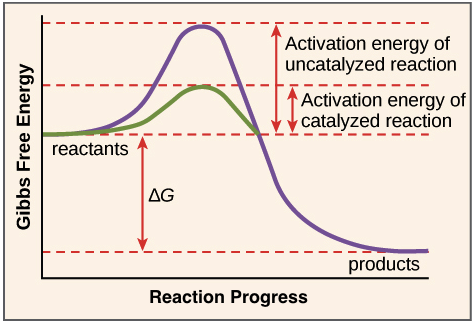# Question f8954

Apr 22, 2017

Enzymes are biological catalysts that speed up the rate of a biochemical reaction without changing the thermodynamics of the system.

#### Explanation:

$\textcolor{b l u e}{\text{Enzymes}}$ are biological catalysts (most of which are proteins) that speed up the rate of a biochemical reaction without changing the thermodynamics of the system, meaning, enzymes will change how fast a reaction occurs (kinetics) and NOT if a reaction occurs(thermodynamics)

$\textcolor{w h i t e}{a a a a a a a}$

$\textcolor{w h i t e}{a a a a a}$How exactly do enzymes lower the activation energy?

In order to understand how enzymes catalyze reactions, we must first get through some basic terminology.

$- - - - - - - - - - - - - - - - - - - - -$The figure above is a $\textcolor{b l u e}{\text{free energy diagram}}$. It shows us the reaction progress (x axis) and the change in $\textcolor{b l u e}{\text{Gibbs Free Energy} \left(\Delta G\right)}$ associated with the reaction (y axis).

$\left(\Delta G\right)$ is just a term scientists used to describe if a reaction is spontaneous or not, or in other words, if the reaction will occur by itself without any added energy.

$\textcolor{w h i t e}{a a a a a a a a}$$\textcolor{red}{\left(-\right) \Delta G \to \text{exergonic reaction" ("spontaneous}}$
$\textcolor{w h i t e}{a a a a a a a a}$$\textcolor{red}{\left(+\right) \Delta G \to \text{endergonic reaction"("nonspontaneous}}$

In the reaction showcased above, the starting point for the reactant, or the $\textcolor{b l u e}{\text{Ground State}}$, is at a higher Gibbs free energy level than the product. This means that the product is thermodynamically more stable than the reactant, and thus, the reaction proceeds spontaneously in the forward direction (exergonic reaction). The $\left(\Delta G\right)$ of the reaction can be calculated as follows:

$\textcolor{w h i t e}{a a a a a a a a a a a a}$$\textcolor{red}{\Delta G = \Delta {G}_{\text{product")–DeltaG_("reactants}}}$

When an $\textcolor{b l u e}{\text{substrate}}$ binds to the enzyme's $\textcolor{b l u e}{\text{active site}}$, together they form an $\textcolor{b l u e}{\text{enzyme-substrate complex [ES]}}$ and subsequent catalysis forms products and regenerates the enzyme.

$\textcolor{w h i t e}{a a a a a a a a a a a a}$$\textcolor{red}{E + S r i g h t \le f t h a r p \infty n s E S \to E + P}$

Before the reaction proceeds, though, it must overcome a certain energy barrier, or $\textcolor{b l u e}{\text{Activation energy}}$. Activation energy, is the change in free energy between the $\text{Ground state}$ and the color(blue)("Transition state"#, the top most energy hill in the diagram.

The $\text{Transition state}$ is a moment in time where bond breakage, bond reformation and the correct orientation for molecules to react occurs. For this to occur, however, sufficient energy is required. So how does an enzyme achieve this?

During enzyme-substrate binding, $\textcolor{b l u e}{\text{weak non-covalent interactions (H bonding, ionic interactions, hydrophobic interactions)}}$ will form which will not only add to the stability of the $\text{[ES]}$ complex but also release some energy called the $\textcolor{b l u e}{\text{Binding energy}}$. Since the energy released by the weak interactions (binding energy) offsets the energy required to reach the transition state, the overall net activation energy is lowered and the reaction can proceed at a faster rate.